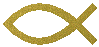MOBILE HOME          SKIRTING    For mobile homes, porches, utility buildings         & how to measure for it. Even if you just need pieces we can do that too. If the steps below are to hard to do then call or email the store with the figures from step one and step three. The computer can handle it. And we can give you an accurate price. If you have leftovers, keep them clean and return within two weeks and get a refund.  1. Measure the length and width of the home. Add these together and double it. Write this figure down in feet to the next largest foot. 2. Divide this figure by 12. Then round up 1. This will give you the total number of trim panels needed. (Top inner and outer and bottom rail.) The same number of top front, back rails and bottom rails will be needed.  3. Measure the four corners in inches. Add these figures together. Divide that figure by 4 and add 4 inches. Divide that figure by 12. That will give you the average height in feet of each panel and account for some deviation in the slope of the ground. Write this figure down in feet.  4. Divide 11.5 feet by the average height that you got in step 3. This will give you the average number of panels per main panel.. 5. Now take the total length (allowing for porches too, if you are going to cover them), in feet, and divide by 1.4. 6. Then divide the resulting number in step five and divide by the average you got from step three. This will give you the average total number of main panels that you will need to cover the outside. I usually add 4 main panels for safety.